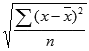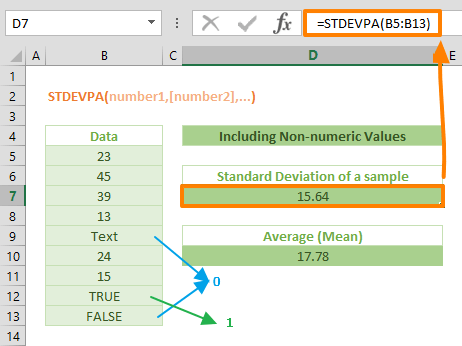The STDEVPA is a Statistical function that calculates and returns the standard deviation for the entire population of data. The standard deviation is a measure of how widely values are dispersed from the average value (the mean). The STDEVPA calculates standard deviation using the "n" method. In this guide, we’re going to show you how to use the STDEVPA function and also go over some tips and error handling methods.

## Supported versions

• All Excel version

## STDEVPA Function Syntax

STDEVPA(number1,[number2],...)

## Arguments

 number1 The first number or reference in the sample population. You can also use an array instead of multiple arguments. The function evaluates text and logical values using thefollowing rules: TRUE = 1 FALSE = 0 Any string = 0 [number2], … Other numbers of the population, up to 254 arguments.

## STDEVPA Function's Example

The standard deviation is a measure expressing by how much the members of a group differ from the mean (average) value for the group. The STDEVPA function assumes that given arguments are the entire population. The function uses the following formula:where x is the average (mean) and n is the sample size.

All you need to do is to enter your data set into the arguments.

=STDEVPA(B5:B13)## Tips

• Use the STDEVPA function to calculate the standard deviation for entire population by taking non-numeric values into calculation.
• The standard deviation is calculated using the "n" method.
• Prefer STDEV.S to calculate the standard deviation for a sample of the population. The STDEV.S function uses "n - 1" method.
• You can use the AVERAGE function to calculate the mean of a data set.
• Arguments can either be numbers or names, arrays, or references that contain numbers.
• Logical values, and text representations of numbers that you type directly into the list of arguments are counted.
• If an argument is an array or reference, only numbers in that array or reference are counted. Empty cells, logical values, text, or error values in the array or reference are ignored.
• Arguments that are error values or text that cannot be translated into numbers cause errors.
• If you want to include logical values and text representations of numbers in a reference as part of the calculation, use the STDEVA function.